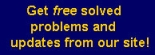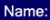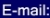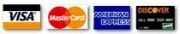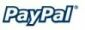# The place to find MATH HOMEWORK HELP online

For a list of free math problems, click on the link (see the snapshot below)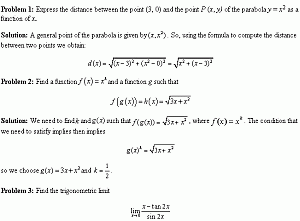Here we provide you with list of solved math problems, for you to use.

Problem 1

Problem 2

Problem 3

Problem 4

Problem 5

Problem 6

Problem 7

Problem 8

Problem 9

Problem 10

Problem 11

Problem 12

Problem 13

Problem 14

Problem 15

Problem 16

Problem 17

 The ways you can submit your problems to us for a FREE quote are: By e-mail: You send us an e-mail with your questions. It can be any type of file, doc, pdf, xls, or a scanned image of the problems. The turnaround is 24-48 hours. By fax: We'll give you our fax number on request.

MGT - Your source of Math and Statistics Homework Help

 Statistics Help   |   Calculus Homework Online   |   Operations Management Help   |   Request a Quote     |     Calculus II and III     |    Linear Algebra     |    Statistical Analysis - Statistics Solutions Contact us at [email protected] © 2005-2017 MyGeekyTutor.com - Your preferred help with math homework All rights reserved